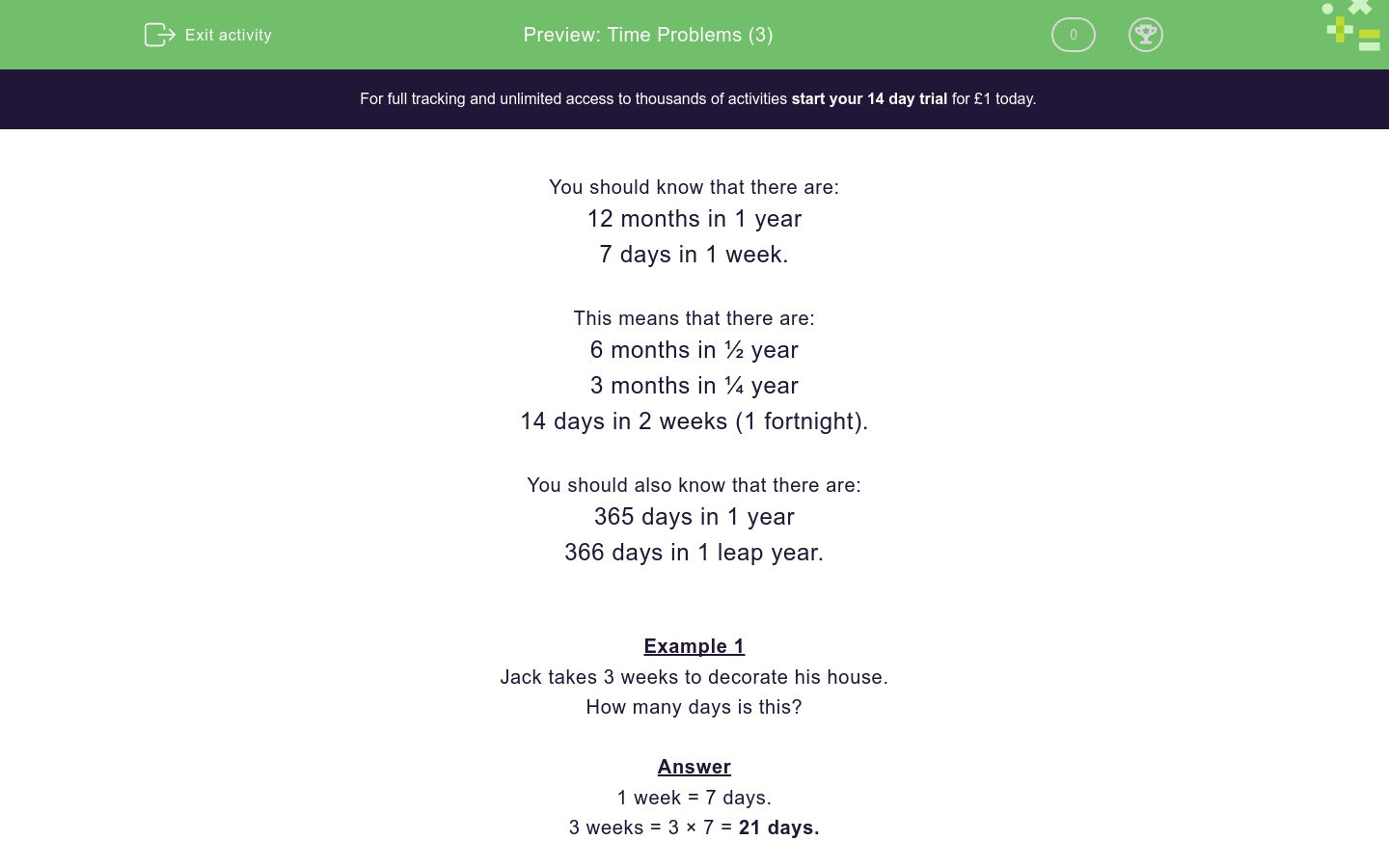# Time Problems (3)

In this worksheet, students must know how many months, weeks and days there are in various time periods, including halves and quarters.Key stage:  KS 2

Curriculum topic:   Measurement

Curriculum subtopic:   Solve Time Problems

Difficulty level:### QUESTION 1 of 10

You should know that there are:

12 months in 1 year

7 days in 1 week.

This means that there are:

6 months in ½ year

3 months in ¼ year

14 days in 2 weeks (1 fortnight).

You should also know that there are:

365 days in 1 year

366 days in 1 leap year.

Example 1

Jack takes 3 weeks to decorate his house.

How many days is this?

1 week = 7 days.

3 weeks = 3 × 7 = 21 days.

Example 2

Annie stays at her job for 4½ years.

How many months is this?

1 year = 12 months.

½ year = 6 months.

4½ years = 4 × 12 + 6 = 48 + 6 = 54 months.

Write the missing number below:

There aredays in 4 weeks.

Write the missing number below:

There aremonths in 2 years.

Write the missing number below:

There aredays in 10 weeks.

Write the missing number below:

There aredays in 2 fortnights.

Choose the missing number below:

18 months is the same asyears.

3

Jack gets 5 weeks of holiday every year.

How many days is this?

Just write the number.

Ann starts building a wall on January 3rd and finishes on January 17th.

How many weeks did she take?

Just write the number.

2

3

14

Write the missing number below:

There aremonths in 1¼ years.

Jack is in hospital for 56 days.

How many weeks is this?

Just write the number.

Baby Aled goes for an injection when he is 30 months old.

How old is he in years?

3 years

2¼ years

2½ years

• Question 1

Write the missing number below:

There aredays in 4 weeks.

28
EDDIE SAYS
7 days = 1 week
4 × 7 = 28
• Question 2

Write the missing number below:

There aremonths in 2 years.

24
EDDIE SAYS
12 months = 1 year
2 × 12 = 24
• Question 3

Write the missing number below:

There aredays in 10 weeks.

70
EDDIE SAYS
7 days = 1 week
10 × 7 = 70
• Question 4

Write the missing number below:

There aredays in 2 fortnights.

28
EDDIE SAYS
1 fortnight = 2 weeks
2 fortnights = 4 weeks
7 × 4 = 28 days
• Question 5

Choose the missing number below:

18 months is the same asyears.

EDDIE SAYS
12 months = 1 year
6 months = ½ year
18 months = 1½ years
• Question 6

Jack gets 5 weeks of holiday every year.

How many days is this?

Just write the number.

35
EDDIE SAYS
1 week = 7 days
5 × 7 = 35 days
• Question 7

Ann starts building a wall on January 3rd and finishes on January 17th.

How many weeks did she take?

Just write the number.

2
EDDIE SAYS
17 - 3 = 14 days
14 days = 2 weeks
• Question 8

Write the missing number below:

There aremonths in 1¼ years.

15
EDDIE SAYS
¼ year = 12 ÷ 4 = 3 months
12 + 3 = 15 months
• Question 9

Jack is in hospital for 56 days.

How many weeks is this?

Just write the number.

8
EDDIE SAYS
1 week = 7 days
56 ÷ 7 = 8 weeks
• Question 10

Baby Aled goes for an injection when he is 30 months old.

How old is he in years?

2½ years
EDDIE SAYS
6 months = ½ year
24 + 6 = 30 months
This is 2 years + ½ year = 2½ years.
---- OR ----

Sign up for a £1 trial so you can track and measure your child's progress on this activity.

### What is EdPlace?

We're your National Curriculum aligned online education content provider helping each child succeed in English, maths and science from year 1 to GCSE. With an EdPlace account you’ll be able to track and measure progress, helping each child achieve their best. We build confidence and attainment by personalising each child’s learning at a level that suits them.

Get started